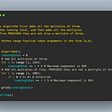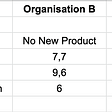# Card Games: Calculating the odds that you have the best card

Various card games require you to predict whether a given card in your hand is the strongest of its suit out of all the cards that have been dealt. Sure, if you have the King of Spades in a game of few players each with a small hand, it’s a fairly safe bet that it’s the strongest spade. But what if there are more players and larger hands? How do we actually calculate the odds that your card is the strongest?

# The Game

To answer this question, let’s consider a simple game in which

1. Each player is dealt an equal number of cards.
2. (Optionally) Bets are placed by each player if they choose— please gamble responsibly.
3. Whichever player has the highest spade is declared the winner and the game ends.

What you want to know is: After the cards are dealt, what are the odds that the strongest spade in your hand is the strongest of all spades that have been dealt?

For those just interested in a cheat sheet, let’s get that out of the way :) The following table describes the odds that a given spade in your hand is the strongest of those dealt, given the card (K=King, Q=Queen, J=Jack, etc.), the number of players in the game, and the size of each player’s hand. Note that Ace is always 100%, and therefore not shown.

Disclaimer: Use this information benevolently — card games are meant to be fun :)

Let’s now explore the formula we used to calculate these odds.

# The Formula

Given the following attributes to describe a card game:

• p: number of players in the game
• n: number of cards in each hand
• R: rank of the card in question, where 0 is best (Ace for Ace-high games), then 1 (King), then 2 (Queen), etc.

The following formula produces the odds (as a percentage) that the card in question is the strongest of those dealt, assuming:

• There are no stronger cards of this suit already in your hand.
• The total number of cards dealt (n * p) is less than or equal to 52.

Let’s break down this formula, which is finding the odds that all cards stronger than the card in question are in the undealt pile.

• The numerator represents the number of cards in the undealt pile.
• The denominator represents the number of cards not known to you, i.e. every card that’s not in your hand.
• The product symbol (capital “pi”) represents that this calculation must be repeated for each value of k from k=0 to k=R-1, i.e. for each rank that is stronger than the card in question, and that the final result is the product of all iterations, i.e. they must all be multiplied together.

# Examples

Let’s consider you’re playing a game with 5 players (including yourself), each with 3 cards. One of your cards is the King of Spades — what are the odds that this is the strongest spade out of those that have been dealt? Using the formula with p=5, n=3, R=1:

Thanks to WolframAlpha for facilitating this calculation and all others in this article.

The result shows that, in this game, there’s an approximately 75% chance that your King of Spades is the strongest spade that’s been dealt. Suppose instead you had the Queen of Spades in this game — what would be the odds of that being the strongest spade? Using the same formula with p=5, n=3, R=2, the odds are 57%.

Let’s also observe how these odds change with the number of players and hand size. The following graph illustrates the odds that a Jack of Spades in your hand is the best spade that’s been dealt when playing a game with p players each having n cards.

In games of few players and small hand sizes, the Jack of Spades will likely be the strongest spade, with 94% odds in the case of 2 players each with 1 card. As p and n increase however, these odds drop, with less than 4% odds of it being the strongest in the case of 6 players each with 6 cards. We can also compare how the changes in p and n affect different cards in the following graph, which compares Ace (which is always 100%), King, Queen, Jack, and 10.

# Simulation

We can also test these odds by simulating games. The following Python code randomly deals n cards each to p players (including yourself), ensuring that you get the card of rank R and no stronger card in your hand. It repeats this over many iterations and counts how often this card was the strongest of those dealt.

`import randomdef play_game(p, n, R):  total_card_count = 52  undealt_cards = range(total_card_count)  your_cards = []  opponent_cards = []  your_cards.append(undealt_cards.pop(R))  # take card in question  dealt_card_target = n*p  dealt_card_count = 1  while dealt_card_count < dealt_card_target:    rand_index = random.randint(0, len(undealt_cards)-1)      card = undealt_cards.pop(rand_index)    if len(your_cards) < n:      if card < R:  # don't accept any stronger cards        undealt_cards.append(card)        continue      your_cards.append(card)    else:      opponent_cards.append(card)    dealt_card_count +=1  for rank in range(R):  # check if any stronger cards in opponents    if rank in opponent_cards:      return False  # card is not strongest  return True  # card is strongestdef simulate(p, n, R, iterations):  print('playing %d games of p: %d, n: %d, R: %d' % (iterations, p, n, R))  strongest = 0  for i in range(iterations):    strongest += 1 if play_game(p, n, R) else 0  print('-> card rank %d is strongest in %.1f%% of games' % (R, 100.0*strongest/iterations))simulate(5, 3, 1, 100000)  # Kingsimulate(5, 3, 2, 100000)  # Queensimulate(2, 1, 3, 100000)  # Jacksimulate(6, 6, 3, 100000)  # Jack`

This program simulates 100,000 games for various values of p, n, and R. The following is output from a run of this program, which closely matches with values previously calculated with the formula.

`playing 100000 games of p: 5, n: 3, R: 1-> card rank 1 is strongest in 75.5% of gamesplaying 100000 games of p: 5, n: 3, R: 2-> card rank 2 is strongest in 56.7% of gamesplaying 100000 games of p: 2, n: 1, R: 3-> card rank 3 is strongest in 94.1% of gamesplaying 100000 games of p: 6, n: 6, R: 3-> card rank 3 is strongest in 3.8% of games`

# Summary

Calculating the odds that a given card in your hand is the strongest of its suit out of all that have been dealt is achieved through a short formula that depends on the number of players in the game, the size of each hand, and the rank of the card in question. Knowing these odds can give you a significant edge in card games that require you to estimate them.

--

--

--

## More from Shubham Sakhuja

Working @ aramse.io, delivering cloud-native solutions to help companies streamline DevOps

Love podcasts or audiobooks? Learn on the go with our new app.

## Multiple Linear Regression in Python: King County Housing Data## Data-science Series (Visual Programming with orange tool):## WEEK 0 EXPERIENCE AT 10ACADEMY## Understanding Annotation Graphs## Automatically Detect COVID-19 Misinformation## All about Graph Data Structure..## Data Visualization in 3 Steps## Shubham Sakhuja

Working @ aramse.io, delivering cloud-native solutions to help companies streamline DevOps

## [Python Tutorial] Project Euler #1: Multiples of 3 or 5## Maximin Strategy in Game Theory## Expensive — Fun.